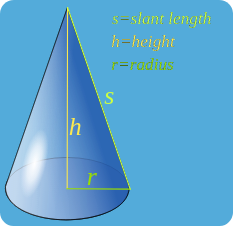# Surface Area of a Cone

##Formula:

With a cone, there are 2 surfaces.

• Curved Surface = π·r·s

• Circular Base = π·r2

For lateral surface area you just need to use the formula for the curved surface.

For total surface area you will need to find the lateral surface area and add the area of the circular base.

## Calculating slant height:

Surface area of a cone will require you to use the slant height instead of the height which forms a 90 degree angle with the base. If you are given only “h” and “r” then you will need to calculate the slant height with Pythagorean theorem:

a2+b2=c2

After replacing the variables:

r2+h2=s2

## Practice:

If a cone has a height of 7 inches and a radius of 2. What is the surface area?

Area of the Base:

πr2=4π=12.57

Surface Area of Side:

First we need to find the slant height:  72+22=s2

s=√(53)

SA=2π√(53)

=45.74

Total Surface Area=12.57+45.74=58.31inches